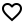## android-demo-app

Project Url: pytorch/android-demo-appIntroduction: PyTorch android examples of usage in applications
More: Author   ReportBugs
Tags:

HelloWorld is a simple image classification application that demonstrates how to use PyTorch Android API. This application runs TorchScript serialized TorchVision pretrained resnet18 model on static image which is packaged inside the app as android asset.

#### 1. Model Preparation

Let’s start with model preparation. If you are familiar with PyTorch, you probably should already know how to train and save your model. In case you don’t, we are going to use a pre-trained image classification model(Resnet18), which is packaged in TorchVision. To install it, run the command below:

``````pip install torchvision
``````

To serialize the model you can use python script in the root folder of HelloWorld app:

``````import torch
import torchvision

model = torchvision.models.resnet18(pretrained=True)
model.eval()
example = torch.rand(1, 3, 224, 224)
traced_script_module = torch.jit.trace(model, example)
traced_script_module.save("app/src/main/assets/model.pt")
``````

If everything works well, we should have our model - `model.pt` generated in the assets folder of android application. That will be packaged inside android application as `asset` and can be used on the device.

More details about TorchScript you can find in tutorials on pytorch.org

#### 2. Cloning from github

``````git clone https://github.com/pytorch/android-demo-app.git
cd HelloWorldApp
``````

If Android SDK and Android NDK are already installed you can install this application to the connected android device or emulator with:

``````./gradlew installDebug
``````

We recommend you to open this project in Android Studio 3.5.1+ (At the moment PyTorch Android and demo applications use android gradle plugin of version 3.5.0, which is supported only by Android Studio version 3.5.1 and higher), in that case you will be able to install Android NDK and Android SDK using Android Studio UI.

``````repositories {
jcenter()
}

dependencies {
implementation 'org.pytorch:pytorch_android:1.3.0'
implementation 'org.pytorch:pytorch_android_torchvision:1.3.0'
}
``````

Where `org.pytorch:pytorch_android` is the main dependency with PyTorch Android API, including libtorch native library for all 4 android abis (armeabi-v7a, arm64-v8a, x86, x86_64). Further in this doc you can find how to rebuild it only for specific list of android abis.

`org.pytorch:pytorch_android_torchvision` - additional library with utility functions for converting `android.media.Image` and `android.graphics.Bitmap` to tensors.

#### 4. Reading image from Android Asset

All the logic happens in `org.pytorch.helloworld.MainActivity`. As a first step we read `image.jpg` to `android.graphics.Bitmap` using the standard Android API.

``````Bitmap bitmap = BitmapFactory.decodeStream(getAssets().open("image.jpg"));
``````

``````Module module = Module.load(assetFilePath(this, "model.pt"));
``````

`org.pytorch.Module` represents `torch::jit::script::Module` that can be loaded with `load` method specifying file path to the serialized to file model.

#### 6. Preparing Input

``````Tensor inputTensor = TensorImageUtils.bitmapToFloat32Tensor(bitmap,
TensorImageUtils.TORCHVISION_NORM_MEAN_RGB, TensorImageUtils.TORCHVISION_NORM_STD_RGB);
``````

`org.pytorch.torchvision.TensorImageUtils` is part of `org.pytorch:pytorch_android_torchvision` library. The `TensorImageUtils#bitmapToFloat32Tensor` method creates tensors in the torchvision format using `android.graphics.Bitmap` as a source.

All pre-trained models expect input images normalized in the same way, i.e. mini-batches of 3-channel RGB images of shape (3 x H x W), where H and W are expected to be at least 224. The images have to be loaded in to a range of `[0, 1]` and then normalized using `mean = [0.485, 0.456, 0.406]` and `std = [0.229, 0.224, 0.225]`

`inputTensor`'s shape is `1x3xHxW`, where `H` and `W` are bitmap height and width appropriately.

#### 7. Run Inference

``````Tensor outputTensor = module.forward(IValue.from(inputTensor)).toTensor();
float[] scores = outputTensor.getDataAsFloatArray();
``````

`org.pytorch.Module.forward` method runs loaded module's `forward` method and gets result as `org.pytorch.Tensor` outputTensor with shape `1x1000`.

#### 8. Processing results

Its content is retrieved using `org.pytorch.Tensor.getDataAsFloatArray()` method that returns java array of floats with scores for every image net class.

After that we just find index with maximum score and retrieve predicted class name from `ImageNetClasses.IMAGENET_CLASSES` array that contains all ImageNet classes.

``````float maxScore = -Float.MAX_VALUE;
int maxScoreIdx = -1;
for (int i = 0; i < scores.length; i++) {
if (scores[i] > maxScore) {
maxScore = scores[i];
maxScoreIdx = i;
}
}
String className = ImageNetClasses.IMAGENET_CLASSES[maxScoreIdx];
``````

In the following sections you can find detailed explanations of PyTorch Android API, code walk through for a bigger demo application, implementation details of the API, how to customize and build it from source.

## PyTorch Demo Application

We have also created another more complex PyTorch Android demo application that does image classification from camera output and text classification in the same github repo.

To get device camera output it uses Android CameraX API. All the logic that works with CameraX is separated to `org.pytorch.demo.vision.AbstractCameraXActivity` class.

``````void setupCameraX() {
final PreviewConfig previewConfig = new PreviewConfig.Builder().build();
final Preview preview = new Preview(previewConfig);
preview.setOnPreviewOutputUpdateListener(output -> mTextureView.setSurfaceTexture(output.getSurfaceTexture()));

final ImageAnalysisConfig imageAnalysisConfig =
new ImageAnalysisConfig.Builder()
.setTargetResolution(new Size(224, 224))
.setCallbackHandler(mBackgroundHandler)
.build();
final ImageAnalysis imageAnalysis = new ImageAnalysis(imageAnalysisConfig);
imageAnalysis.setAnalyzer(
(image, rotationDegrees) -> {
analyzeImage(image, rotationDegrees);
});

CameraX.bindToLifecycle(this, preview, imageAnalysis);
}

void analyzeImage(android.media.Image, int rotationDegrees)
``````

Where the `analyzeImage` method process the camera output, `android.media.Image`.

It uses the aforementioned `TensorImageUtils.imageYUV420CenterCropToFloat32Tensor` method to convert `android.media.Image` in `YUV420` format to input tensor.

After getting predicted scores from the model it finds top K classes with the highest scores and shows on the UI.

#### Language Processing Example

Another example is natural language processing, based on an LSTM model, trained on a reddit comments dataset. The logic happens in `TextClassificattionActivity`.

Result class names are packaged inside the TorchScript model and initialized just after initial module initialization. The module has a `get_classes` method that returns `List[str]`, which can be called using method `Module.runMethod(methodName)`:

``````    mModule = Module.load(moduleFileAbsoluteFilePath);
IValue getClassesOutput = mModule.runMethod("get_classes");
``````

The returned `IValue` can be converted to java array of `IValue` using `IValue.toList()` and processed to an array of strings using `IValue.toStr()`:

``````    IValue[] classesListIValue = getClassesOutput.toList();
String[] moduleClasses = new String[classesListIValue.length];
int i = 0;
for (IValue iv : classesListIValue) {
moduleClasses[i++] = iv.toStr();
}
``````

Entered text is converted to java array of bytes with `UTF-8` encoding. `Tensor.fromBlobUnsigned` creates tensor of `dtype=uint8` from that array of bytes.

``````    byte[] bytes = text.getBytes(Charset.forName("UTF-8"));
final long[] shape = new long[]{1, bytes.length};
final Tensor inputTensor = Tensor.fromBlobUnsigned(bytes, shape);
``````

Running inference of the model is similar to previous examples:

``````Tensor outputTensor = mModule.forward(IValue.from(inputTensor)).toTensor()
``````

After that, the code processes the output, finding classes with the highest scores.

Apps